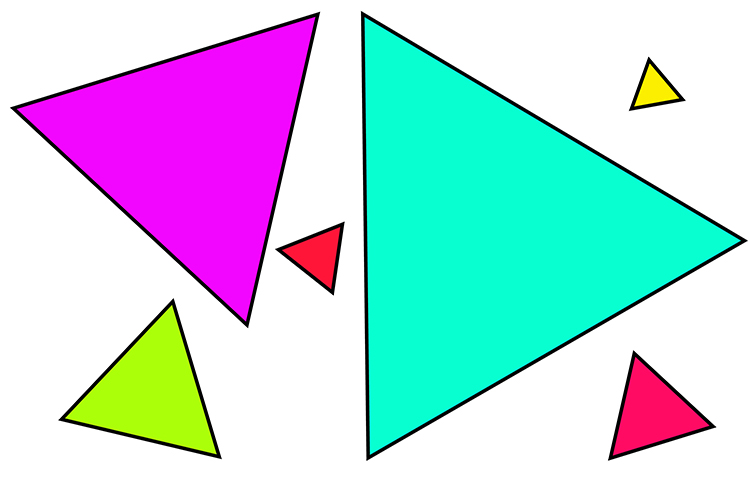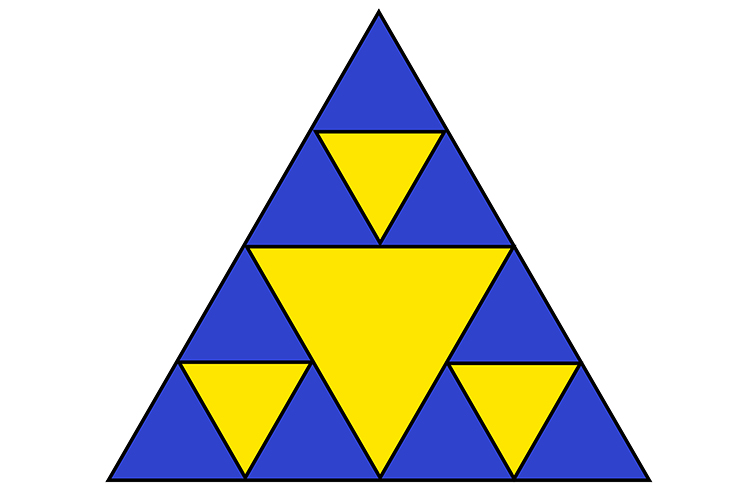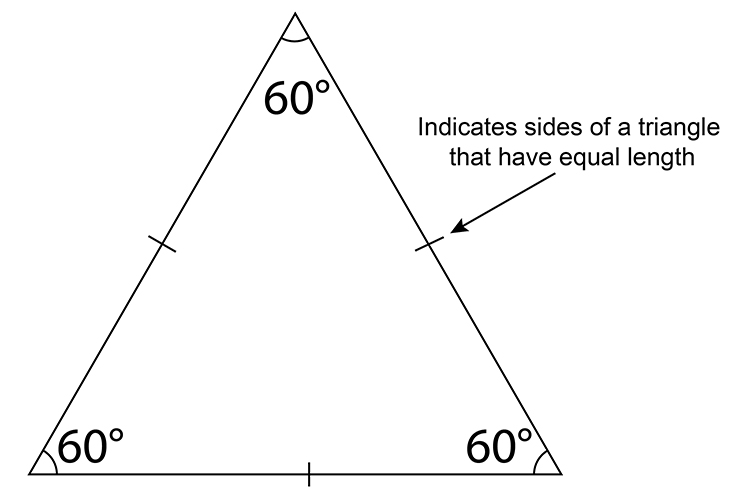# Equilateral

Equilateral triangle is one in which all three sides have same length.

Equilateral starts with the word EQUAL

Equilateral starts with the word equal (or the starts have similar sounds) to remind you that all sides of the triangle are equal.

ExamplesDifferent sized equilateral trianglesThis triangle is made up of many triangles, but all of them are equilateral.All the sides are equal length and all the internal angles are 60° on an equilateral triangle.

NOTE:

The internal angle between each of an equilateral triangles sides is always 60°.